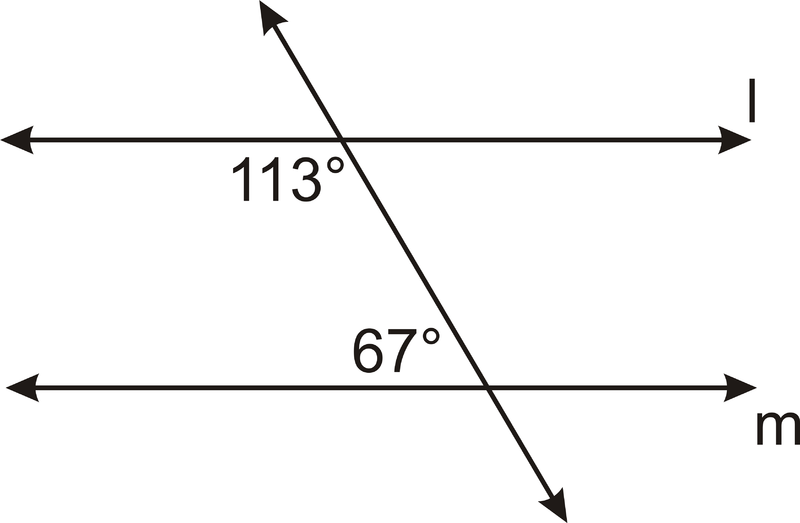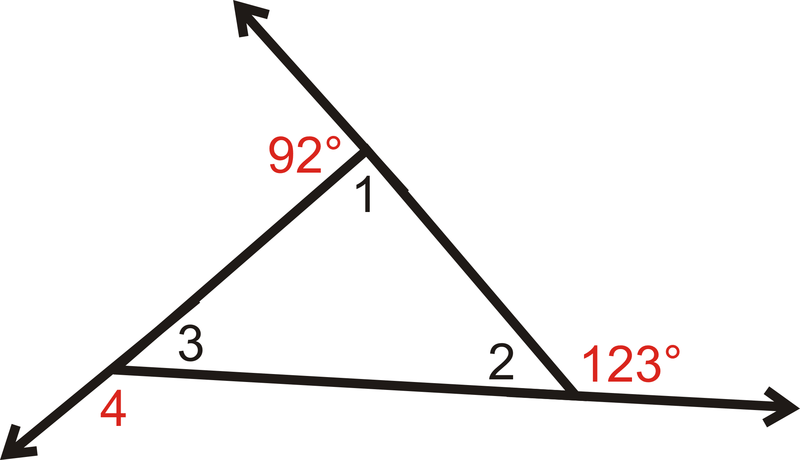# Parallel linesIn geometryparallel lines are lines in a plane which do not meet; that is, two lines in a plane that do not intersect or touch each other at any point are said to be parallel. By extension, a line and a plane, or two planes, in three-dimensional Euclidean space that do not share a point are said to be parallel. However, two lines in three-dimensional space which do not meet must be in a common plane to be considered parallel; otherwise they are called skew lines. Parallel planes are planes in the same three-dimensional space that never meet.

Parallel lines are the subject of Euclid‘s parallel postulate. Parallelism is primarily a property of affine geometries and Euclidean geometry is a special instance of this type of geometry. In some other geometries, such as hyperbolic geometry, lines can have analogous properties that are referred to as parallelism.

# Interior anglegeometry, an angle of a polygon is formed by two sides of the polygon that share an endpoint. For a simple (non-self-intersecting) polygon, regardless of whether it is convex or non-convex, this angle is called an interior angle (or internal angle) if a point within the angle is in the interior of the polygon. A polygon has exactly one internal angle per vertex.

If every internal angle of a simple polygon is less than 180°, the polygon is called convex.

# Exterior anglean exterior angle (or external angle) is an angle formed by one side of a simple polygon and a line extended from an adjacent side.:pp. 261-264